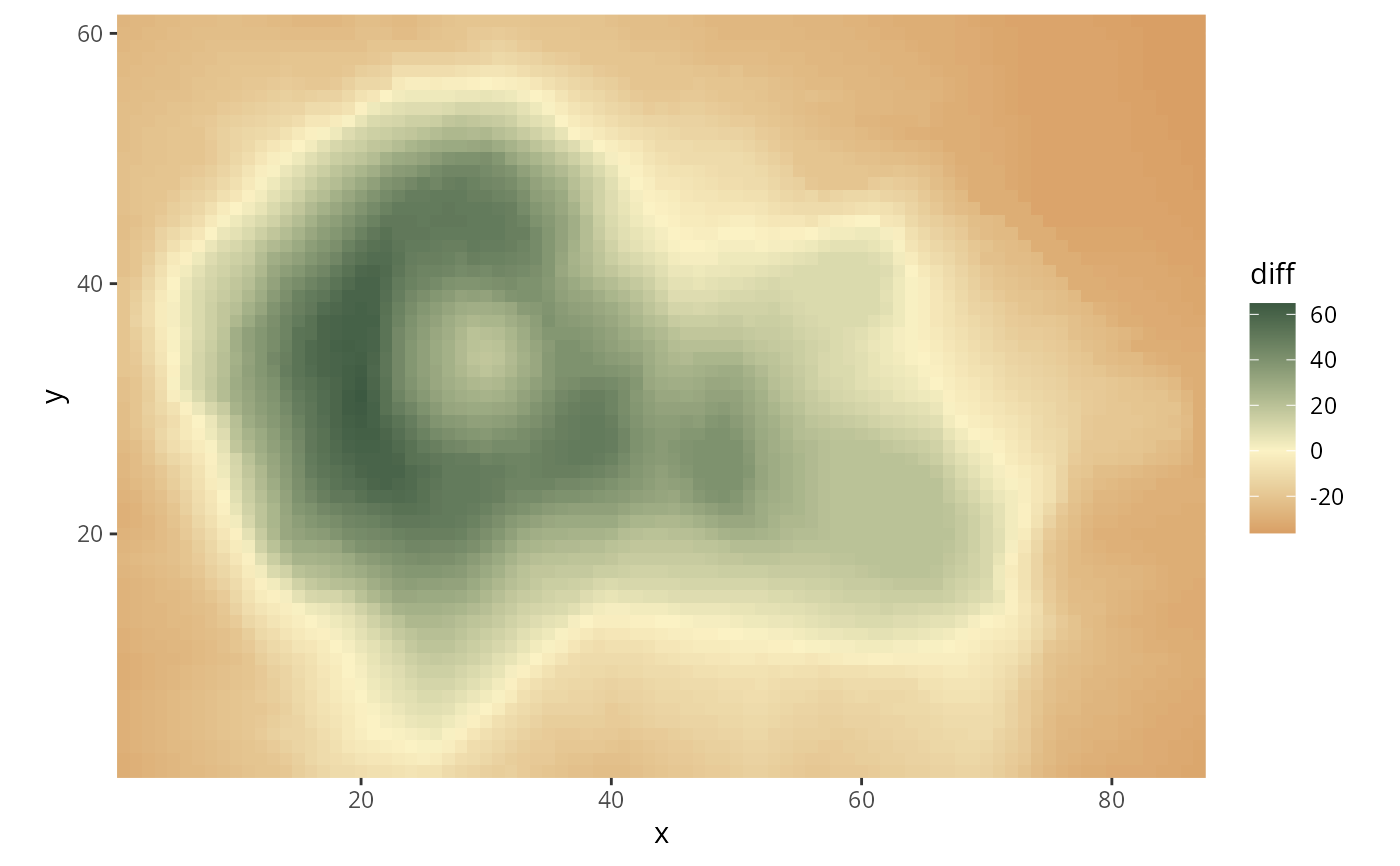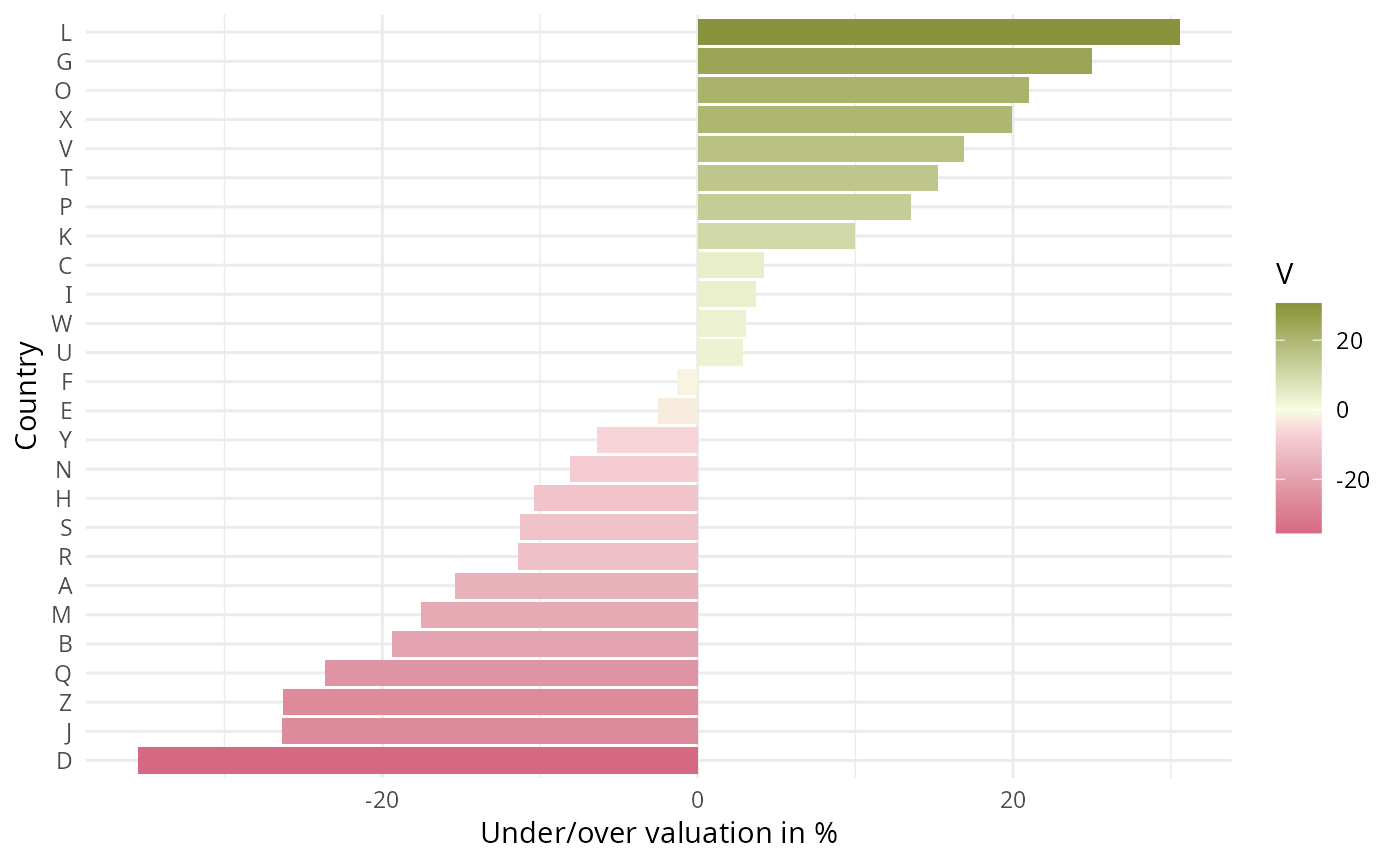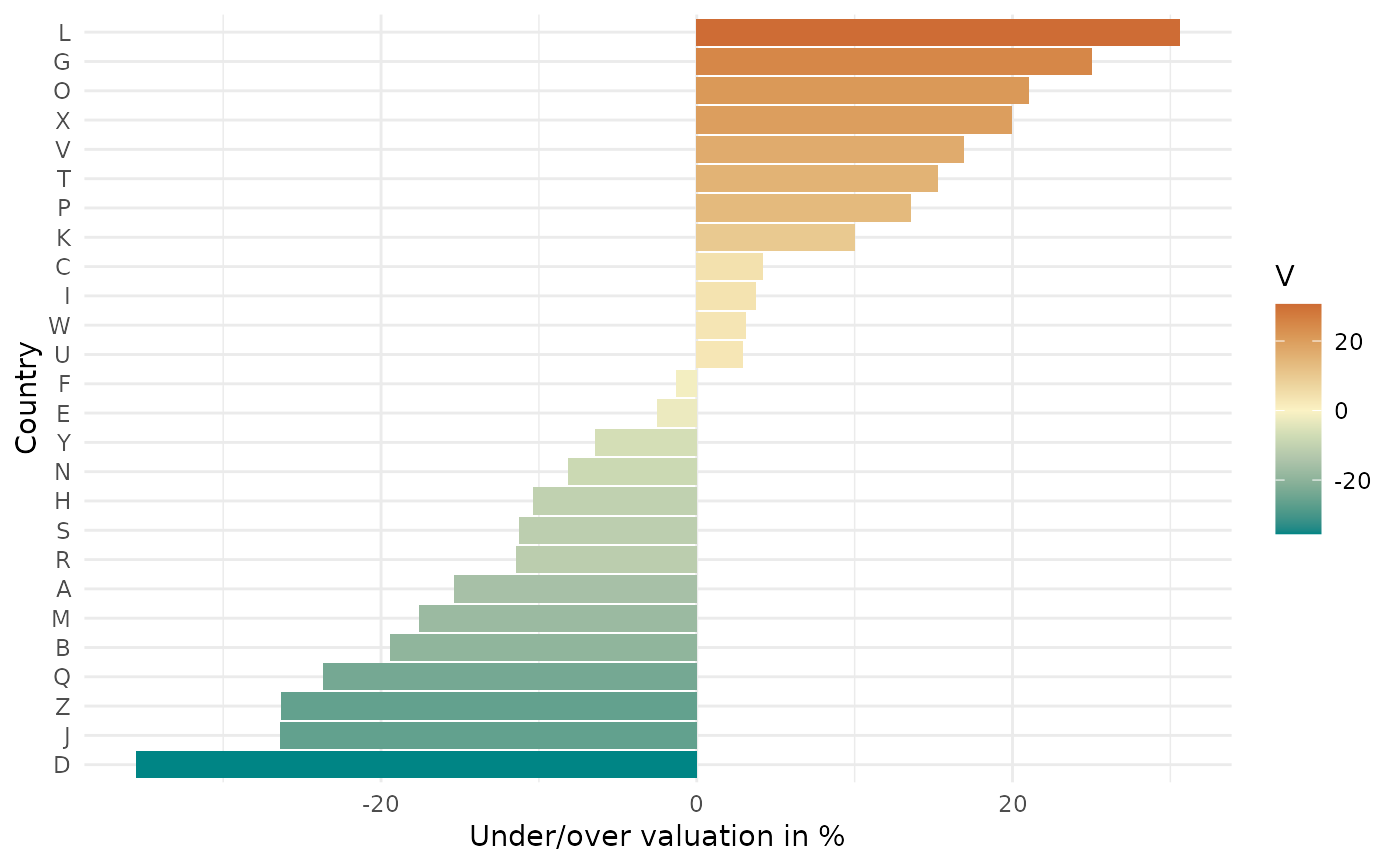Continuous ggplot2 color scales using the color palettes generated by divergingx_hcl.

scale_colour_continuous_divergingx(
palette = "Geyser",
c1 = NULL,
c2 = NULL,
c3 = NULL,
l1 = NULL,
l2 = NULL,
l3 = NULL,
h1 = NULL,
h2 = NULL,
h3 = NULL,
p1 = NULL,
p2 = NULL,
p3 = NULL,
p4 = NULL,
cmax1 = NULL,
cmax2 = NULL,
rev = FALSE,
begin = 0,
end = 1,
mid = 0,
na.value = "grey50",
guide = "colourbar",
n_interp = 11,
aesthetics = "colour",
...
)

scale_color_continuous_divergingx(
palette = "Geyser",
c1 = NULL,
c2 = NULL,
c3 = NULL,
l1 = NULL,
l2 = NULL,
l3 = NULL,
h1 = NULL,
h2 = NULL,
h3 = NULL,
p1 = NULL,
p2 = NULL,
p3 = NULL,
p4 = NULL,
cmax1 = NULL,
cmax2 = NULL,
rev = FALSE,
begin = 0,
end = 1,
mid = 0,
na.value = "grey50",
guide = "colourbar",
n_interp = 11,
aesthetics = "colour",
...
)

scale_fill_continuous_divergingx(..., aesthetics = "fill")

## Arguments

palette The name of the palette to be used. Parameters to customize the scale. See divergingx_hcl for details. If TRUE, reverses the order of the colors in the color scale. For sequential scales, number in the range of [0, 1] indicating to which point in the color scale the smallest data value should be mapped. Ignored for diverging scales. For sequential scales, number in the range of [0, 1] indicating to which point in the color scale the largest data value should be mapped. Ignored for diverging scales. For diverging scales, data value that should be mapped to the mid-point of the diverging color scale. Ignored for sequential scales. Color to be used for missing data points. Type of legend. Use "colourbar" for continuous color bar. Number of discrete colors that should be used to interpolate the continuous color scale. For diverging scales, it is important to use an odd number to capture the color at the midpoint. The ggplot2 aesthetics to which this scale should be applied. common continuous scale parameters: name, breaks, labels, and limits. See continuous_scale for more details.

## Details

Available CARTO palettes: ArmyRose, Earth, Fall, Geyser, TealRose, Temps, Tropic.

Available ColorBrewer.org palettes: Spectral, PuOr, RdYlGn, RdYlBu, RdGy, BrBG, PiYG, PRGn, RdBu.

If both a valid palette name and palette parameters are provided then the provided palette parameters overwrite the parameters in the named palette. This enables easy customization of named palettes.

## Examples

# *** Examples for sequential CARTO scales ***

# base plot
library("ggplot2")
gg <- ggplot(iris, aes(x = Species, y = Sepal.Width, color = Sepal.Length)) +
geom_jitter(width = 0.3) + theme_minimal()

# default settings
gg + scale_color_continuous_divergingx()# switch palette to ArmyRose
gg + scale_color_continuous_divergingx(palette = "ArmyRose")# select a range out of the entire palette
gg + scale_color_continuous_divergingx(palette = "ArmyRose", begin = .2, end = .8)# volcano plot
nx = 87
ny = 61
df <- data.frame(height = c(volcano), x = rep(1:nx, ny), y = rep(1:ny, each = nx))
ggplot(df, aes(x, y, fill=height)) +
geom_raster() + scale_fill_continuous_divergingx(palette = "Fall", rev = TRUE) +
coord_fixed(expand = FALSE)# *** Examples for diverging CARTO scales ***

# generate dataset and base plot
library("ggplot2")
set.seed(100)
df <- data.frame(country = LETTERS, V = runif(26, -40, 40))
df$country = factor(LETTERS, LETTERS[order(df$V)]) # reorder factors
gg <- ggplot(df, aes(x = country, y = V, fill = V)) +
geom_bar(stat = "identity") +
labs(y = "Under/over valuation in %", x = "Country") +
coord_flip() + theme_minimal()

# plot with diverging scale "Geyser"
gg + scale_fill_continuous_divergingx(palette = "Geyser")# plot with diverging scale "ArmyRose"
gg + scale_fill_continuous_divergingx(palette = "ArmyRose")